# Diamond Turning Ogive Error and the Implications for Optical Tolerancing

The quality of machined optical surfaces has drastically improved, thanks to the latest advances in diamond turning lathes. However, conditions that are not related to the geometry of the machine tool significantly contribute to surface form errors, including cutting tool conditions, improper component mounting, and dynamic machining effects.

Hence, gaining insights into these conditions is crucial in order to achieve further significant improvements in terms of the quality of directly machined surfaces. This article discusses one aspect of these conditions and its effect on the machined optical surface. This aspect is associated with diamond machining with tool offsets, and its effect on surface distortion is termed as ogive error.

## Ogive Geometrical Model

The term ‘ogive’ is related to a gothic architectural design form. Figure 1 shows the constructional aspects of an ogive form. The generating curve is the basis of an ogive form and is generally a circular arc section. The generating curve is positioned symmetrically about the axis of the ogive figure to form the figure.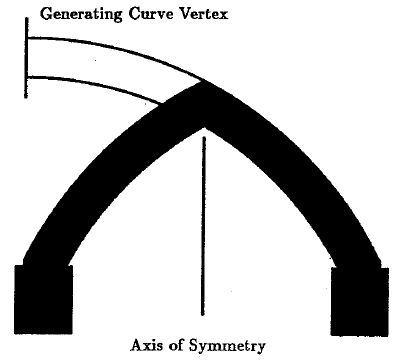Figure 1. Ogive arch

The degree of "pointedness" of the ogive figure relies on the degree of offset between the axis of symmetry and the generating curve vertex. It is to be noted that the vertex can lie on both sides of the axis forming open and closed ogive figures, like in two-axis diamond machining, where the cutting tool is offset from the centerline of the rotating workpiece.

The two-axis orthogonal diamond machining involves movement of the diamond tool in relation to a rotating workpiece in a coordinated motion along two axes that are at right angles to describe a generating curve in space. Tool offset must be there if the tool is not synchronized with the rotational axis of the workpiece at the vertex of the generating curve.

A machined surface created with a tool offset is defined as an ogive surface of revolution. This definition will provide the physical basis for this model of diamond turning ogive error. This model is simplified based on the following assumptions:

• The coordinated motion of the tool in describing the generating curve is ideal
• The rotating workpiece and the centerline for the generating figure are parallel
• Tool radius effects are compensated
• Dynamic machining effects are absent

The Pythagorean model for ogive geometry is presented in Figure 2. Offset is shown by the vertex of the generating curve offset from the centerline of a coordinate system, which describes the rotating workpiece by the amounts δx and δy.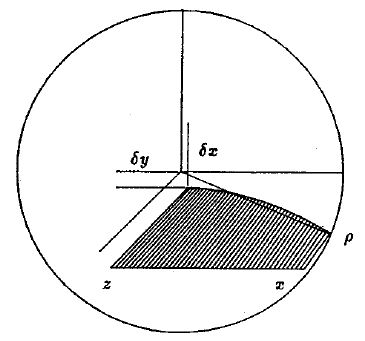Figure 2. Ogive geometry

Pythagorean sum of the tool offsets and the radial distance, x1, on the generating curve is the distance from the rotating centerline to a cutting point on the curve and surface (ρ).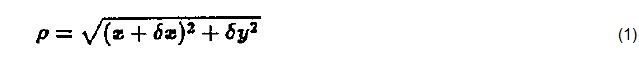It is possible to invert Equation 1 to yield the radial coordinate in the machining plane as a function of the rotational coordinate.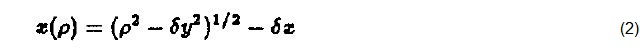If the generating curve is characterized as a sagittal function of the radial coordinate, i.e. z = z(x), then the ogive surface of revolution is expressed by a direct substitution with Equation 2.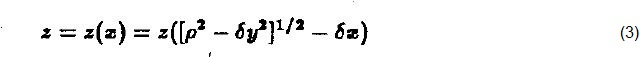The case of an ogive "sphere" is first investigated prior to the application of this Pythagorean representation for ogive error to general aspheric surfaces. This is crucial due to the possibility of calculating and correcting the amount of tool offset present to a degree through the evaluation of a "sphere" with ogive error.

For a sphere, the generating curve is a circular section. Equation for an ogive "sphere" can be derived by making a direct substitution from Equation 3 using the common sagitta equation for the circular section with ‘r’ radius.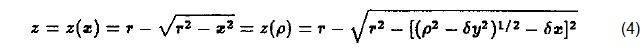The manner by which the tool offset δx enters this equation is different from the tool offset δy. A binomial expansion of Equation 2 can be derived by assuming that the rotational coordinate is much larger than the tool offsets. This hypothesis is correct over a major part of the surface, except at the center of rotation, where the geometry will be dominated by the diamond tool radius in the presence of a δx offset, and a cosmetic defect due to a δy offset.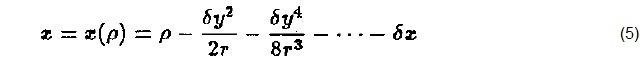As shown in Equation 5, the influence of δy offsets on the ogive figure is less than δx offsets over the remainder of the surface due to the relative strength of the terms. As a result, the height of diamond tools can be set with the help of a microscope and there is a need for an interferometer for reduction of x offsets. Moreover, δx is sign dependent but δy is present in an even power expansion in the equation.

In addition, it is not possible to differentiate from the macroscopic ogive surface when a tool offset in the y direction positions the tool above or below rotational centerline. However, it is comparatively simple to identify which side of the rotational axis the vertex of the generating curve lies. Equation 5 takes a differential form when the terms with higher order offset dependencies are neglected.This simplification provides the equation for the sagittal variation between this ogive "sphere" and the same sphere without an offset.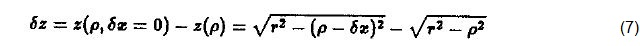If it is assumed that the sphere radius is much higher than the surface aperture, binomial expansion of these radicals is possible.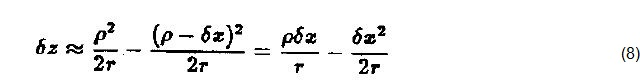The following sagitta difference is obtained when the terms with higher order offset dependences are neglected.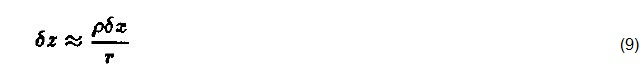In the rotational coordinate (ρ), the profile of an ogive "sphere" is a conical error profile. It is possible to displace the center of the reference wavefront in the interferometric determination of such a surface so that the conical error appears tilted. The selection of an appropriate displacement allows the optical path difference to be minimized between the center of the figure and one edge, resulting in double the sagitta error between the center and the opposite edge. The δx offset from an interferometric evaluation of the ogive "sphere" can be determined from an inversion of Equation 9 along with this factor of two from the tilted profile.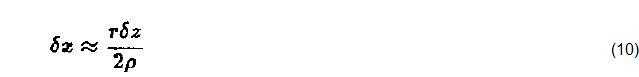This relationship is illustrated in Figure 3, which shows a synthetic interferogram produced from a ray-trace algorithm with rays traced off the following surface using the exact representation of the ogive surface as expressed in Equation 4. The optical path difference between the left-hand edge and center is minimized by the proper displacement of the reference spherical wavefront center, allowing to observe the expected four fringes at 632.8 nm, or 1.25 µm of δz.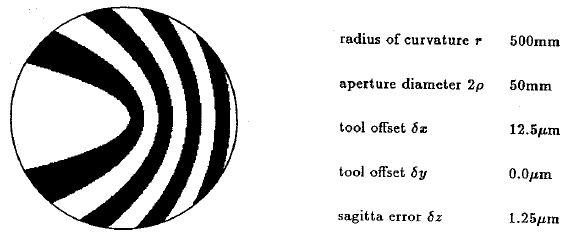Figure 3. Synthetic inteferogram for an ogive “sphere”

## Ogive Form Errors on Aspheric Surfaces

Equation 11 is a widely used sagitta equation applied for representing aspheric optical surfaces, defining a conic surface of revolution altered by simple polynomial terms.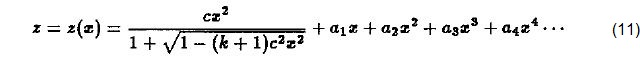The ogive form for the aspheric surface is derived by a direct substitution from Equation 2. It is possible to obtain optical tolerancing for ogive errors by ray-tracing using this representation. The approximations of Equation 6 help achieving an in-depth analysis of optical tolerancing. If this expression is considered to be an exact differential, it is possible to obtain a simplified representation of the sagitta difference as a product of the derivative of the aspheric generating curve and the δz offset.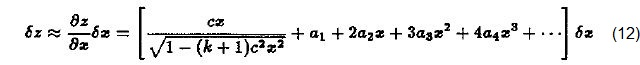This means that ogive form error is influenced by the slope of the generating equation, but not by the total surface sagitta. The slope is based on higher order polynomial terms or the optical speed of the surface. Based on this simplification, it is possible to make certain observations. Ogive error does not affect a linear axicon or a cone shaped surface, which will, however, suffer from an axial displacement of the surface. For a parabolic surface, the ogive error will have a linear radial dependence. This is in good agreement with the approximations made for the case of an ogive "sphere".

Equation 12 has the same form as the radial shearing interferometer equation in which the differential (δx) is the displacement between the radially sheared wavefronts. Aspheric surfaces are often evaluated using radial shearing interferometers. However, the similarity between the testing technique and the ogive surface poses a challenge to make the correct interpretation of the interference pattern. Hence, this effect has to be considered in the evaluation of diamond turned surfaces using radial shearing interferometry.

## Tolerancing Considerations and Ogive Error

Surface distortions in an optical system have a complex relationship with image quality. The impact of surface distortions on the image quality is contributed to by factors such as wavefront obliquity, field angle considerations, surface proximity to stops and pupils, and surrounding media. Hence, the tolerancing of optical surfaces to meet the imaging requirement of a system must consider these factors and more. The combination of ray-tracing and the Pythagorean model can be used to tolerance surfaces with ogive error in order to obtain exact simulations. It is possible to analyze tolerancing considerations of ogive error by studying the influence on image quality of wavefronts aberrated by ogive surfaces.

Besides being radially symmetric, ogive aberrated wavefronts would be related to the derivative of the defining aspheric generating curve. The effects of different order aspheric terms on image quality can be compared using wavefronts composed of simple radial dependent terms. The wavefront error (δw) is expressed as follows: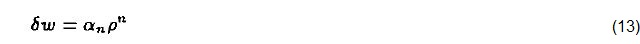where αn is a coefficient corresponding to the severity of the ogive error.

The Strehl intensity for such a system will be deteriorated by the square of the root-mean-square departure from a reference spherical wavefront in the case of having small wavefront errors. rms values for wavefronts are compared with different radial dependencies, but with the same peak-to-valley error to obtain a measure of the sensitivity of these wavefront errors. It is possible to calculate the rms values for these wavefronts from the following definition: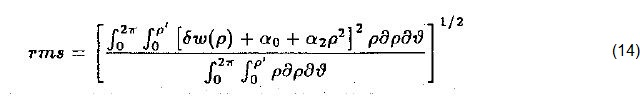Equation 14 calculates the rms value based on the wavefront error (δw), a best fit piston term (α0), and a best fit defocus term (α2). It is possible to simplify the analysis by considering the integration over a unit circle exit pupil (i.e. p' = 1) and with an unfocused peak-to-valley wavefront error of unity (i.e. α1 = 1).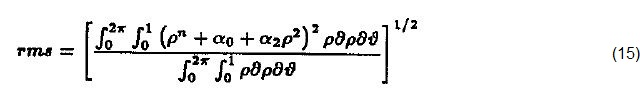Equation 16 expresses the rms following the integration: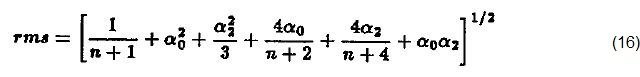It is possible to take partial derivatives of Equation 16, and determine optimized values for piston (α0) and defocus (α2) algebraically from a least squares reduction.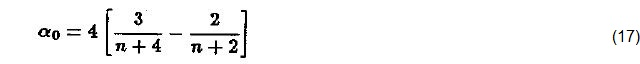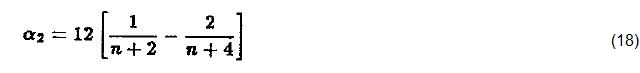It is possible to calculate the values for the peak-to-valley and rms wavefront distortions by substituting these optimized piston and defocus values into Equation 16. Table 1 summarizes the results:

Table 1. Wavefront data for different polynomial terms

exponent α0 α2 P - V RMS
1 -0.266667 -0.800000 0.312500 0.047140
2 0.000000 -1.000000 0.000000 0.000000
3 0.114286 -1.028571 0.161213 0.042857
4 0.166667 -1.000000 0.250000 0.074536
5 0.190476 -0.952381 0.347910 0.097202
6 0.200000 -0.900000 0.428634 0.113389
7 0.202020 -0.848485 0.495347 0.124994
8 0.200000 -0.800000 0.550882 0.133333
9 0.195804 -0.755245 0.597515 0.139317
10 0.190476 -0.714286 0.637022 0.143577

In this example, a parabolic reflector is considered as the cause for the differences in wavefront rms values for aberrated wavefront. The power dependence is first-order if the wavefront error is aberrated owing to ogive error. The power dependence is fourth-order if the wavefront error is analogous to third-order spherical aberration. Table 1 shows that the ogive error effect on rms is nearly half the effect of third-order spherical aberration (i.e. 0.047140 to 0.074536).

The Strehl ratio, and therefore the image quality of a system, will be considerably better for the ogive paraboloid than the spherically aberrated paraboloid. In this case, optical tolerancing of the ogive surface can be significantly loosened in comparison with a model for spherically aberrated surface distortion. Synthetic interferograms for these wavefronts are presented in Figure 4.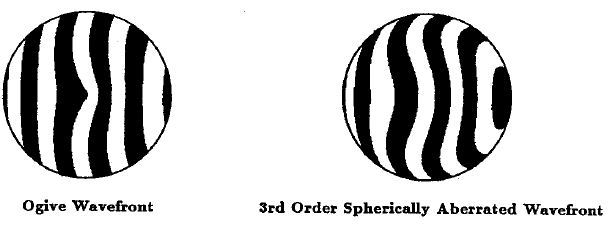Figure 4. Synthetic Interferogram for Aberrated Wavefronts

## Conclusion

This article has demonstrated the application of diamond machining ogive error on a Pythagorean model for tool offset. The investigation of the form error of an ogive "sphere" and asphere has been performed using this model. The results also show the dependency of optical tolerancing for ogive error on the derivative of the aspheric surface form.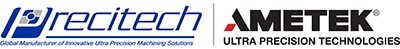This information has been sourced, reviewed and adapted from materials provided by Precitech.

## Citations

• APA

AMETEK Precitech. (2019, December 12). Diamond Turning Ogive Error and the Implications for Optical Tolerancing. AZoM. Retrieved on October 23, 2020 from https://www.azom.com/article.aspx?ArticleID=11924.

• MLA

AMETEK Precitech. "Diamond Turning Ogive Error and the Implications for Optical Tolerancing". AZoM. 23 October 2020. <https://www.azom.com/article.aspx?ArticleID=11924>.

• Chicago

AMETEK Precitech. "Diamond Turning Ogive Error and the Implications for Optical Tolerancing". AZoM. https://www.azom.com/article.aspx?ArticleID=11924. (accessed October 23, 2020).

• Harvard

AMETEK Precitech. 2019. Diamond Turning Ogive Error and the Implications for Optical Tolerancing. AZoM, viewed 23 October 2020, https://www.azom.com/article.aspx?ArticleID=11924.